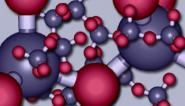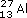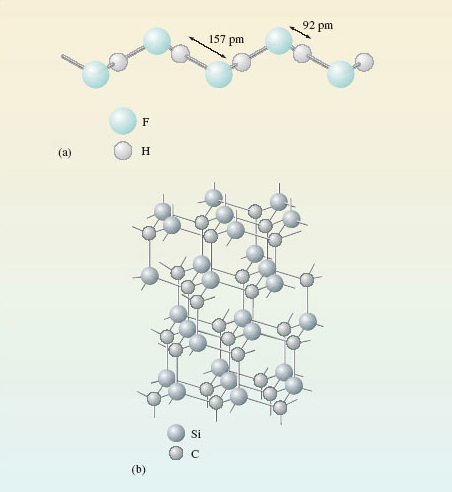Science, Maths & Technology

### Become an OU studentThe molecular world

Start this free course now. Just create an account and sign in. Enrol and complete the course for a free statement of participation or digital badge if available.

# 1.7 Summary of Section 1

• All materials are made of atoms of about 120 different chemical elements, each element being characterised by an atomic number which lies in the range 1-120.

• Each atom has a nucleus where most of its mass resides. The atomic number is equal to the number of units of positive charge on the nucleus, the number of protons in the nucleus, and to the number of surrounding electrons in the neutral atom.

• The nuclei of nearly all atoms contain neutrons as well as protons. The mass number of an atom is the sum of the numbers of neutrons and protons.

• All atoms of an element contain the same number of protons, but they may differ in the number of neutrons. This gives rise to atoms of an element with the same atomic number but different mass numbers. These different types of atom are called isotopes. In chemical changes isotopes behave almost identically.

• Chemical compounds are combinations of the atoms of two or more chemical elements. The empirical formula of a compound tells us the ratio in which the atoms of its elements are combined.

• Molecular substances have structures from which discrete molecules can be picked out by using interatomic distance as a criterion; non-molecular substances do not. The formula of these discrete molecules is called the molecular formula. Most molecular compounds contain just one type of molecule, which then has the same empirical formula as the compound. Most organic compounds are molecular substances.

• Molecular substances usually have lower melting and boiling temperatures than non-molecular ones. They also tend to dissolve more easily in organic solvents such as petrol.

## Question 1

The element aluminium (symbol Al; atomic number 13) has a relative atomic mass of 27.0; in nature it contains just one isotope.

• (a) State the number of protons in the aluminium nucleus.

• (b) State the number of orbiting electrons around the nucleus of an aluminium atom.

• (c) If the electronic charge is written −e, what is the total charge on the nucleus of the aluminium atom?

• (d) State the number of neutrons in the aluminium nucleus.

• (e) Write down the isotope that is present in natural aluminium by combining mass number, atomic number and chemical symbol.

(a) 13;

(b) 13;

(c) +13e;

(d) 14;

(e). Because the atomic number of aluminium is 13, there are 13 protons in the nucleus, 13 orbiting electrons in the atom, and the total charge on the nucleus is + 13e. As the relative atomic mass is 27.0, and there is just one isotope, the mass number is 27. Therefore, the number of neutrons is (27 − 13) = 14. The mass number is the preceding superscript, and the atomic number is the preceding subscript in the symbol.

## Question 2

The molecular formula of an oxide of phosphorus is P4O10.

• (a) What is the empirical formula of the oxide?

• (b) Can the empirical formula of a molecular compound consisting of one type of molecule, contain more atoms than its molecular formula? Explain your answer.

(a) P2O5.

(b) No. The empirical formula can be obtained from the molecular formula by reducing the ratio between the numbers of atoms to the lowest possible whole numbers. A molecule containing fewer atoms than the empirical formula would therefore consist of fractions of atoms. In chemistry, the term 'fraction of an atom' is meaningless.

## Question 3

Figure 15a shows the structure of solid hydrogen fluoride, HF, which consists of zigzag chains containing hydrogen and fluorine atoms. Figure 15b shows the structure of solid silicon carbide, SiC.

• (a) Classify the compounds as molecular or non-molecular.

• (b) One of the compounds (X) melts at −83 °C and is slightly soluble in petrol. The other (Y) remains solid even at 2 500 °C and is insoluble in petrol. Which is which? Explain your answer.Figure 15 The structures of: (a) zigzag chains in solid hydrogen fluoride; (b) solid silicon carbide, in which all distances between atoms linked by lines are 165 pm